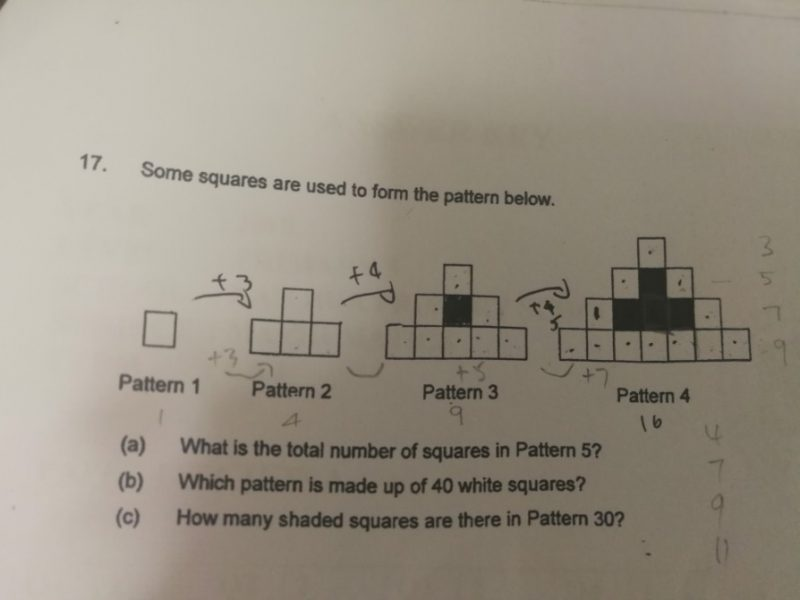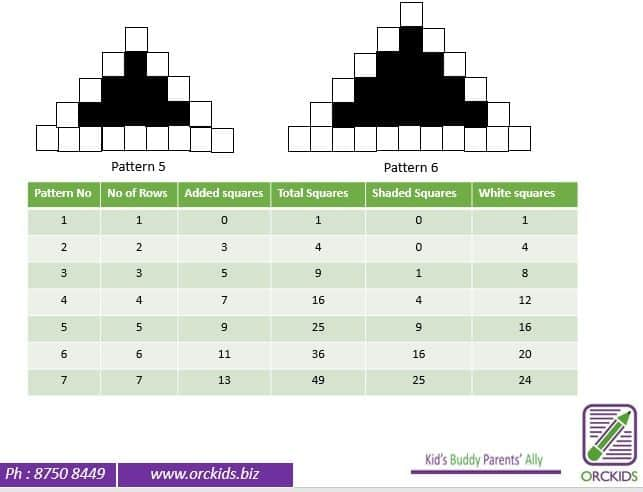# QuestionHi,

Can someone help ?

ThanksNumber of rows in a pattern       = Pattern Number.

New squares added in a pattern = (2 x Pattern Number) – 1

Total squares in pattern                = Pattern Number x Pattern number

Shaded squares in pattern            = (Pattern Number – 2)  x (Pattern Number – 2)

White squares in pattern              = (Pattern Number – 1) x 4

(a) What is the total number of squares in pattern 5?

5 x 5 = 25

(b) Which pattern is made up of 40 white squares?

(11 – 1) x 4 = 40

40

(c) How many shaded squares are there in pattern 30?

(30 – 2) x (30 – 2)

28 x 28 = 784

0 Replies 1 Like